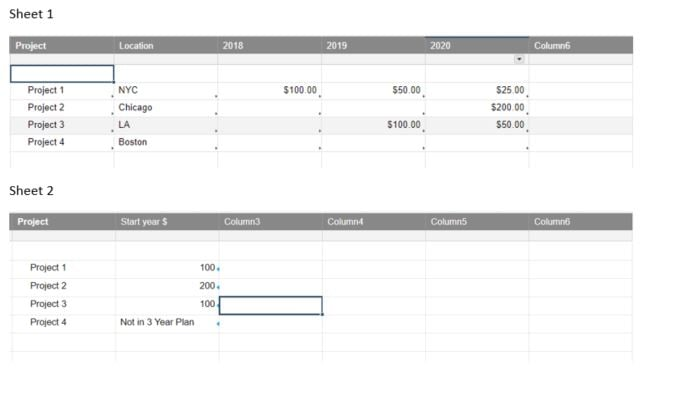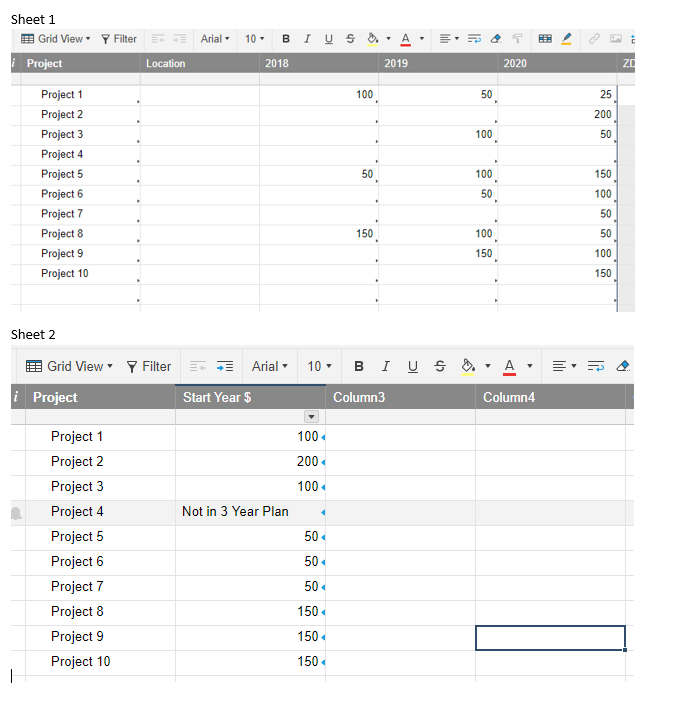# Reference the value of an active cell?

Is there a way to reference the value of an 'active cell value' in a formula or have a moving reference?

The current example code and screenshot specifically call out the desired outcome by referencing each cell in a range.

Example:  Project1 will start in 2018, which is reflected on sheet 2.

formula in column 2 row 2 on sheet 2:

=IF(VLOOKUP(Project2, {SHEET 1 Range 3}, 3, false) > 0, {SHEET 1 Range 2}, IF(VLOOKUP(Project2, {SHEET 1 Range 3}, 4, false) > 0, {SHEET 1 Range 4}, IF(VLOOKUP(Project2, {SHEET 1 Range 3}, 5, false) > 0, {SHEET 1 Range 5}, "Not in 3 Year Plan")))

Is there a way I could get the formula to display the \$ amount of the start year without specifically referencing each cell? I was looking to see if there was an 'active cell' type reference.

Example of thought:

=IF(VLOOKUP(Project2, {SHEET 1 Range 3}, 3, false) > 0, ActiveCell.value, IF(VLOOKUP(Project2, {SHEET 1 Range 3}, 4, false) > 0, ActiveCell.value, IF(VLOOKUP(Project2, {SHEET 1 Range 3}, 5, false) > 0, ActiveCell.value, "Not in 3 Year Plan")))

Any thoughts on this would be a great help. Thank you.• I feel like an INDEX/MATCH may have the flexibility you're looking for. Let me play around with it some, and I'll get back to you...

Are you trying to pull the EARLIEST year populated?

Update: If you are trying to pull the earliest year populated, you could use the following formula updating any cross-sheet references as needed of course.

=IFERROR(INDEX({Community Test Range 1}, MATCH(Project@row, {Community Test Range 2}, 0), COUNTIFS(INDEX({Community Test Range 1}, MATCH(Project@row, {Community Test Range 2}, 0)), ISBLANK(@cell)) + 1), "Not in 3 Year Plan")

See image below for testing of this particular solution.• Yes, I am trying to pull the value from the EARLIEST year populated. Thanks!

• Not sure if you saw it or not, but I have updated my original post with a solution. Hope it helps.

Just a question, what are the cell ranges for the references "Community Test Range 1" and Community Test Range 2"?

• Oops. My apologies...

Range 1 is the year columns. Range 2 is the Project column.

Basically the way it all works is this...

The first portion of the INDEX function establishes where to pull the data from that you are wanting to display which would be your year columns.

The second portion of an INDEX function determines the row. For that we use a MATCH function to look for the Project Name.

The third portion is the column number which is where it got a little tricky. First we re-established the row with the original INDEX/MATCH as we did in parts one and two. Then we counted the number of blank spaces and added 1. This gave us the number of columns to move from left to right to pull the data from.

## Help Article Resources

Want to practice working with formulas directly in Smartsheet?

Check out the Formula Handbook template!